#In mathematics, a fraction is a way of expressing a quantity based on an amount that is divided into a number of equal-sized parts. For example, each part of a cake split into four equal parts is called a quarter (and represented numerically as ¼); two quarters is one half (½) of the cake, and eight quarters would make two cakes.

Mathematically, a fraction is a quotient of numbers, like ¾ (which is 3 divided by 4). More generally, a fraction is an element of a field of fractions.

In our cake example above, where a quarter is represented numerically as ¼, the bottom number, called the denominator, is the total number of equal parts making up the cake as a whole, and the top number, called the numerator, is the number of these parts we have. For example, the fraction ¾ represents three quarters.

The numerator and denominator may be separated by a slanting line called a solidus or slash, for example ¾, or may be written above and below a horizontal line called a vinculum: this is what fractions will look like in most math curriculum.

The numerator and denominator are the "terms" of the fraction. The word "numerator" is related to the word "enumerate," meaning to "tell how many"; thus the numerator tells us how many parts we have in the indicated fraction. To denominate means to "give a name" or "tell what kind"; thus the denominator tells us what kind of parts we have (halves, thirds, fourths, etc.). Note that because it is impossible to divide something into zero equal parts, zero can never be the denominator of a fraction.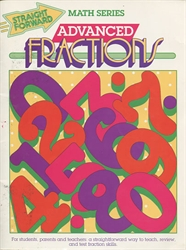Advanced Fractions Straight Forward Math Series by S. Harold Collins from Garlic Press for 3rd-6th grade in Fractions (Location: MATR-FRA) \$2.50 (1 in stock)Cuisenaire Fraction Squares from Cuisenaire Company of America, Inc. in Fractions (Location: MATR-FRA) \$7.50 (1 in stock)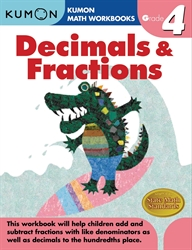Decimals & Fractions Grade 4 Workbook from Kumon Publishing for 4th grade in Kumon Math Skills (Location: MAT-KUM) \$7.95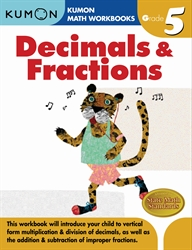Decimals & Fractions Grade 5 Workbook from Kumon Publishing for 5th grade in Kumon Math Skills (Location: MAT-KUM) \$7.95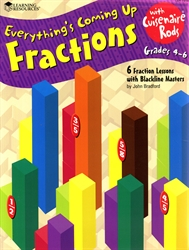Everything's Coming Up Fractions with Cuisenaire Rods by John Bradford from Learning Resources for 4th-6th grade in Cuisenaire Rod Materials (Location: MATM-ROD) \$6.00 (1 in stock)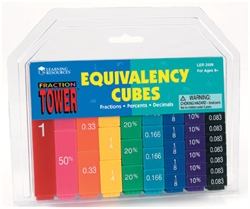Fraction Tower Equivalency Cubes from Learning Resources for 1st-8th grade in Fractions (Location: MATR-FRA) \$19.99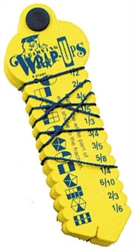Fraction Wrap-Up Keys from Learning Wrap-Ups for 3rd-6th grade in Fractions (Location: MATR-FRA) \$9.99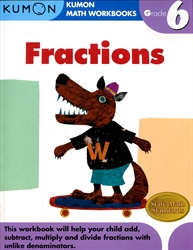Fractions Grade 6 Workbook from Kumon Publishing for 6th grade in Kumon Math Skills (Location: MAT-KUM) \$7.95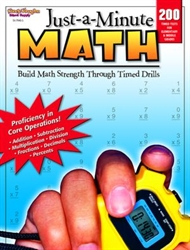Just-a-Minute Math from Steck-Vaughn Company for Kindergarten-4th grade in Math Drill (Location: MATR-DRI) \$19.99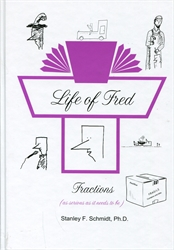Life of Fred: Fractions Life of Fred by Stanley Schmidt from Polka Dot Publishing for 4th-6th grade in Life of Fred Math (Location: MAT-FRED) \$24.00 \$13.00 (2 in stock)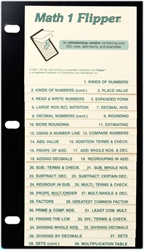Math 1 Flipper from Christopher Lee Publications for 3rd-5th grade in Math Flippers (Location: MATR-FLIP)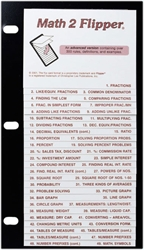Math 2 Flipper from Christopher Lee Publications for 6th-12th grade in Math Flippers (Location: MATR-FLIP)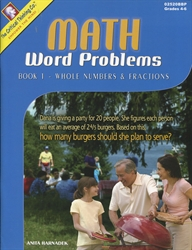Math Word Problems Book 1 by Anita Harnadek from Critical Thinking Books for 4th-6th grade in Critical Thinking Math (Location: MAT-CTP) \$14.99 \$7.50 (2 in stock)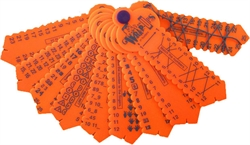Numbers Wrap-Up Keys from Learning Wrap-Ups for 3rd-6th grade in Counters and Counting (Location: MATM-COUN)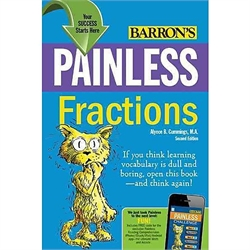Painless Fractions by Alyece Cummings 3rd edition from Barron's for 4th-6th grade in Fractions (Location: MATR-FRA) \$10.99 \$6.00 (1 in stock)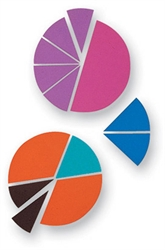Rainbow Fraction Circles from Learning Resources for 1st-8th grade in Fractions (Location: MATR-FRA)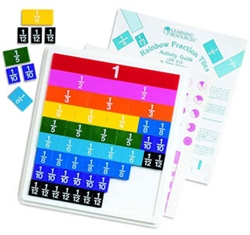Rainbow Fraction Tiles from Learning Resources for 1st-8th grade in Fractions (Location: MATR-FRA) \$13.00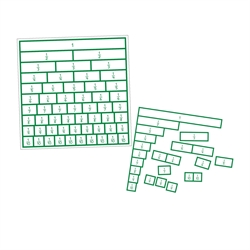RightStart Fraction Activities Chart for 1st-2nd grade in RightStart Mathematics (Location: MAT-RSM) \$13.00 (1 in stock)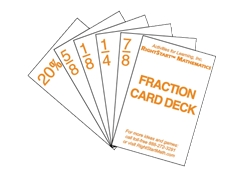RightStart Fraction Card Deck from Activities for Learning, Inc. for Kindergarten-6th grade in RightStart Mathematics (Location: MAT-RSM) \$4.50 (2 in stock)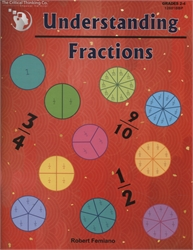Understanding Fractions by Robert Femiano from Critical Thinking Books for 2nd-4th grade in Critical Thinking Math (Location: MAT-CTP) \$13.99